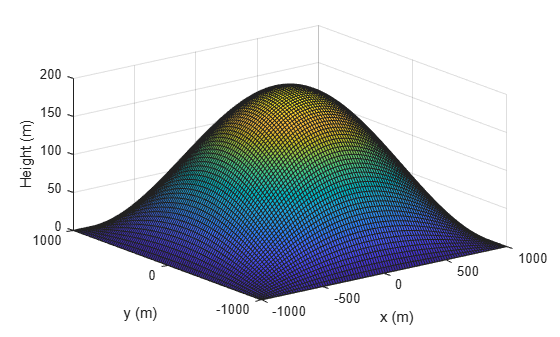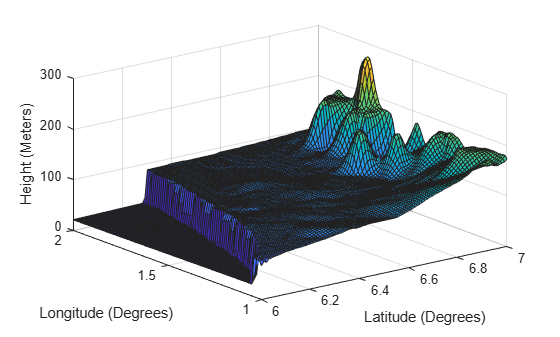# groundSurface

## Syntax

``surface = groundSurface(sc)``
``surface = groundSurface(sc,Name=Value)``

## Description

````surface = groundSurface(sc)` adds a `GroundSurface` object, `surface`, to the tracking scenario `sc`.```

example

````surface = groundSurface(sc,Name=Value)` specifies properties of the created `GroundSurface` object using one or more name-value arguments. For example, `groundSurface(sc,ReferenceHeight=10)` specifies the reference height of the ground surface as 10 meters. Unspecified properties take default values.```

## Examples

collapse all

Create a mesh grid that spans from -1000 meters to 1000 meters in both the x- and y-directions.

`[x,y] = meshgrid(linspace(-1000,1000,500));`

Specify the height for each mesh grid point.

`z = 200*cos(x*pi/2000).*cos(y*pi/2000);`

Create a tracking scenario and add a ground surface object to the tracking scenario. Specify the boundary of the surface area.

```scene = trackingScenario; surface = groundSurface(scene,Terrain=z,Boundary=[-1e3 1e3; -1e3 1e3])```
```surface = GroundSurface with properties: Terrain: [500x500 double] ReferenceHeight: 0 Boundary: [2x2 double] ```

Note that the `SurfaceManager` property of the tracking scenario now contains the created `GroundSurface` object.

`manager = scene.SurfaceManager`
```manager = SurfaceManager with properties: UseOcclusion: 1 Surfaces: [1x1 fusion.scenario.GroundSurface] ```
`manager.Surfaces`
```ans = GroundSurface with properties: Terrain: [500x500 double] ReferenceHeight: 0 Boundary: [2x2 double] ```

Visualize the surface using the `helperGetTerrainMap` helper function, attached to this example.

```xSamples = linspace(-1e3,1e3,100); ySamples = linspace(-1e3,1e3,100); helperGetTerrainMap(surface,xSamples,ySamples); xlabel("x (m)") ylabel("y (m)") zlabel("Height (m)")```Create a tracking scenario and specify its `IsEarthCentered` property as `true`.

`scene = trackingScenario(IsEarthCentered=true);`

Add a ground surface based on a DTED file, covering from 6 to 7 degrees in latitude and from 1 to 2 degrees in longitude.

```terrain = "n06.dt0"; boundary = [6 7; % Latitude in degrees 1 2]; % Longitude in degrees surface = groundSurface(scene,Terrain=terrain,Boundary=boundary);```

Sample the area using a 100-by-100 grid map.

```samples = 100; latitudes = linspace(6,7,samples); longitudes = linspace(1,2,samples); positions = [latitudes; longitudes];```

Plot the terrain using the `helperGetTerrrainMap` helper function, attached to this example`.`

```helperGetTerrainMap(surface,latitudes,longitudes); xlabel("Latitude (Degrees)"); ylabel("Longitude (Degrees)"); zlabel("Height (Meters)");```## Input Arguments

collapse all

Tracking scenario, specified as a `trackingScenario` object.

### Name-Value Arguments

Specify optional pairs of arguments as `Name1=Value1,...,NameN=ValueN`, where `Name` is the argument name and `Value` is the corresponding value. Name-value arguments must appear after other arguments, but the order of the pairs does not matter.

Before R2021a, use commas to separate each name and value, and enclose `Name` in quotes.

Example: `surface = groundSurface(sc,ReferenceHeight=10)`

Terrain data for the surface, specified as an M-by-N real-valued matrix, or a string scalar specifying a Digital Terrain Elevation Data (DTED) file name.

• M-by-N real-valued matrix — The matrix values represent the height data of an area defined by the `Boundary` property of the ground surface object. The object extends the height data in the matrix to the area. The object automatically fills heights of unspecified points using linear interpolation. M and N must both be greater than 3.

• String scalar or character vector specifying DTED file name — To use this option, you must specify the `IsEarthCentered` property of the tracking scenario as `true`. In this case, the object specifies the terrain heights for the area using those defined in the DTED file. The ground surface object automatically fills unspecified data in the DTED file using linear interpolation. If you want to use only a part of the terrain defined in the DTED file, specify the `Boundary` property as your desired subset of the terrain area defined in the DTED file. Otherwise, specify the `Boundary` property as the whole area defined in the DTED file.

Data Types: `single` | `double` | `char`

Boundary of the surface, specified as a 2-by-2 matrix of real values with the form `[xmin xmax; ymin ymax]`. When the `IsEarthCentered` property of tracking scenario object is specified as:

• `false` — Specify `xmin`, `xmax`, `ymin` `ymax`, in meters, as Cartesian coordinates in the reference frame of the scenario, where `xmin` < `xmax`, and `ymin` < `ymax`

• `true` — Specify `xmin` and `xmax` as the minimum and maximum latitudes of the geodetic frame in degrees, where `xmin` < `xmax`. Specify `ymin` and `ymax` as the minimum and maximum longitudes of the geodetic frame in degrees. If `ymax` < `ymin`, the object wraps `ymax` to `ymax` + `360`.

Data Types: `single` | `double`

Reference height of the terrain data, specified as a scalar in meters. Specify the terrain data relative to this reference height.

Data Types: `single` | `double`

## Output Arguments

collapse all

Ground surface, returned as a `GroundSurface` object.

## Version History

Introduced in R2022a